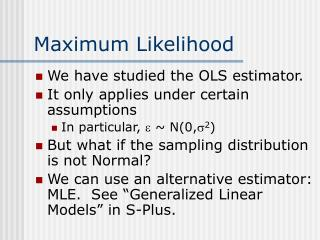DownloadDownload PresentationMaximum Likelihood

# Maximum Likelihood

Télécharger la présentation## Maximum Likelihood

- - - - - - - - - - - - - - - - - - - - - - - - - - - E N D - - - - - - - - - - - - - - - - - - - - - - - - - - -
##### Presentation Transcript

1. Maximum Likelihood • We have studied the OLS estimator. • It only applies under certain assumptions • In particular, e ~ N(0,s2) • But what if the sampling distribution is not Normal? • We can use an alternative estimator: MLE. See “Generalized Linear Models” in S-Plus.

2. OLS vs. MLE • If assumptions of OLS hold, OLS and MLE give exactly same estimates! • So, using MLE instead of OLS is OK! • MLE called “Generalized Linear Models” in S-Plus. • More general than “Linear Regression” • Allows you to specify dist’n of error.

3. Example: Ozone Attainment • “Out of Attainment” if ozone exceeds standard on a given day. • Model distribution of number of days out of attainment in a given county over 20 years. • Use a Poisson Distribution • Estimate the parameter using Maximum Likelihood.

4. MLE • Principle: choose parameter(s) that make observing the given data the most probable (or “likely”). • How do we measure “likelihood”? • If we know sampling distribution, know how “probable” or “likely” any given data are. • So we can measure likelihood. • We must know the distribution.

5. Graph of Likelihood

6. Log-Likelihood • Maximizing log-likelihood is equivalent to maximizing likelihood.

7. Solution • We can model number of exceedences as Poisson distribution. • 1 parameter. • Estimated with maximum likelihood • Estimated parameter (q) is 2.45